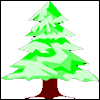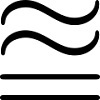# Resources tagged with: Inequalities

Filter by: Content type:
Age range:
Challenge level:

### There are 19 results

Broad Topics > Algebraic expressions, equations and formulae > Inequalities### Not Continued Fractions

##### Age 14 to 18 Challenge Level:

Which rational numbers cannot be written in the form x + 1/(y + 1/z) where x, y and z are integers?### Two Cubes

##### Age 14 to 16 Challenge Level:

Two cubes, each with integral side lengths, have a combined volume equal to the total of the lengths of their edges. How big are the cubes? [If you find a result by 'trial and error' you'll need to. . . .### Square Mean

##### Age 14 to 16 Challenge Level:

Is the mean of the squares of two numbers greater than, or less than, the square of their means?### Rationals Between...

##### Age 14 to 16 Challenge Level:

What fractions can you find between the square roots of 65 and 67?### All-variables Sudoku

##### Age 11 to 18 Challenge Level:

The challenge is to find the values of the variables if you are to solve this Sudoku.### Fracmax

##### Age 14 to 16 Challenge Level:

Find the maximum value of 1/p + 1/q + 1/r where this sum is less than 1 and p, q, and r are positive integers.### Unit Interval

##### Age 14 to 18 Challenge Level:

Take any two numbers between 0 and 1. Prove that the sum of the numbers is always less than one plus their product?### Tet-trouble

##### Age 14 to 16 Challenge Level:

Is it possible to have a tetrahedron whose six edges have lengths 10, 20, 30, 40, 50 and 60 units?### Biggest Enclosure

##### Age 14 to 16 Challenge Level:

Three fences of different lengths form three sides of an enclosure. What arrangement maximises the area?### Inside Outside

##### Age 14 to 16 Challenge Level:

Balance the bar with the three weight on the inside.##### Age 14 to 16 Challenge Level:

Kyle and his teacher disagree about his test score - who is right?### Proofs with Pictures

##### Age 14 to 18

Some diagrammatic 'proofs' of algebraic identities and inequalities.### Approximating Pi

##### Age 14 to 18 Challenge Level:

By inscribing a circle in a square and then a square in a circle find an approximation to pi. By using a hexagon, can you improve on the approximation?### Inequalities

##### Age 11 to 14 Challenge Level:

A bag contains 12 marbles. There are more red than green but green and blue together exceed the reds. The total of yellow and green marbles is more than the total of red and blue. How many of. . . .### Christmas Trees

##### Age 11 to 14 Challenge Level:

Christmas trees are planted in a rectangular array. Which is the taller tree, A or B?### Almost Total Inequality

##### Age 14 to 16 Challenge Level:### Balance Point

##### Age 14 to 16 Challenge Level:

Attach weights of 1, 2, 4, and 8 units to the four attachment points on the bar. Move the bar from side to side until you find a balance point. Is it possible to predict that position?### ' Tis Whole

##### Age 14 to 18 Challenge Level:

Take a few whole numbers away from a triangle number. If you know the mean of the remaining numbers can you find the triangle number and which numbers were removed?### Plutarch's Boxes

##### Age 11 to 14 Challenge Level:

According to Plutarch, the Greeks found all the rectangles with integer sides, whose areas are equal to their perimeters. Can you find them? What rectangular boxes, with integer sides, have. . . .